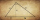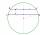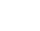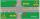# Pythagorean theorem - 9th grade (14y) - math problems

#### Number of problems found: 581

• Total areaCalculate the total area (surface and bases) of a prism whose base is a rhombus which diagonals of 12cm and 18cm and prism height is 10 cm.
• MIT 1869You know the length of hypotenuse parts 9 and 16, at which the hypotenuse of a right triangle is divided by a perpendicular running from its opposite vertex. The task is to find the lengths of the sides of the triangle and the length of the line x. This a
• Two chordsIn a circle with a radius of 8.5 cm, two parallel chords are constructed, the lengths of which are 9 cm and 12 cm. Find the distance of the chords in a circle.
• Digging a pitThe pit has the shape of a regular quadrilateral truncated pyramid. The edges of the bases are 14m and 10m long. The sidewalls form an angle of 135° with a smaller base. Determine how many m3 of soil were excavated when digging the pit?
• Quadrilateral prismThe height of a regular quadrilateral prism is v = 10 cm, the deviation of the body diagonal from the base is 60°. Determine the length of the base edges, the surface, and the volume of the prism.
• Horses playgroundThe fence for the horses has the shape of a rectangular trapezoid with an area of 400 m2, the base lengths should be 31 m and 19 m. How many meters of boards will they need to fence it if the boards are stacked in 5 rows?
• Ratio of sidesCalculate the area of a circle with the same circumference as the circumference of the rectangle inscribed with a circle with a radius of r 9 cm so that its sides are in ratio 2 to 7.
• JourneyCharles and Eva stands in front of his house, Charles went to school south at speed 5.4 km/h, Eva went to the store on a bicycle eastwards at speed 21.6 km/h. How far apart they are after 10 minutes?
• Hexagon rotationA regular hexagon of side 6 cm is rotated through 60° along a line passing through its longest diagonal. What is the volume of the figure thus generated?
• The regularThe regular triangular prism has a base in the shape of an isosceles triangle with a base of 86 mm and 6.4 cm arms, the height of the prism is 24 cm. Calculate its volume.
• Prism 4 sidesThe prism has a square base with a side length of 3 cm. The diagonal of the sidewall of the prism/BG/is 5 cm. Calculate the surface of this prism in cm square and the volume in liters
• Body diagonalCuboid with base 7cm x 3,9cm and body diagonal 9cm long. Find the height of the cuboid and the length of the diagonal of the base,
• Tangent spheresA sphere with a radius of 1 m is placed in the corner of the room. What is the largest sphere size that fits into the corner behind it? Additional info: Two spheres are placed in a corner of a room. The spheres are each tangent to the walls and floor and
• 9-gon pyramidCalculate the volume and the surface of a nine-sided pyramid, the base of which can be inscribed with a circle with radius ρ = 7.2 cm and whose side edge s = 10.9 cm.
• Similarity coefficientThe ratio of similarity of two equilateral triangles is 3.5 (ie 7:2). The length of the side of smaller triangle is 2.4 cm. Calculate the perimeter and area of ​​the larger triangle.
• Triangular prism - regularThe regular triangular prism is 7 cm high. Its base is an equilateral triangle whose height is 3 cm. Calculate the surface and volume of this prism.
• Frustrum - volume, areaCalculate the surface and volume of the truncated cone, the radius of the smaller figure is 4 cm, the height of the cone is 4 cm and the side of the truncated cone is 5 cm.
• SailingSolve the following problem graphically. The fishing boat left the harbor early in the morning and set out to the north. After 12 km of sailing, she changed course and continued 9 km west. Then When she docked and reached the fishing grounds she launched
• ABCD squareIn the ABCD square, the X point lies on the diagonal AC. The length of the XC is three times the length of the AX segment. Point S is the center of the AB side. The length of the AB side is 1 cm. What is the length of the XS segment?
• Triangle SASCalculate the triangle area and perimeter, if the two sides are 51 cm and 110 cm long and angle them clamped is 130 °.

Do you have an interesting mathematical word problem that you can't solve it? Submit a math problem, and we can try to solve it.

We will send a solution to your e-mail address. Solved examples are also published here. Please enter the e-mail correctly and check whether you don't have a full mailbox.

Please do not submit problems from current active competitions such as Mathematical Olympiad, correspondence seminars etc...

Pythagorean theorem is the base for the right triangle calculator. Pythagorean theorem - math word problems. Examples for 9th grade.